0%

# 课堂笔记

## 人脸识别

### 单样本学习

1. 每来一个新同事，模型就要重新训练一次。
2. 每个人都得上传大量的个人照片供网络训练。

### 孪生网络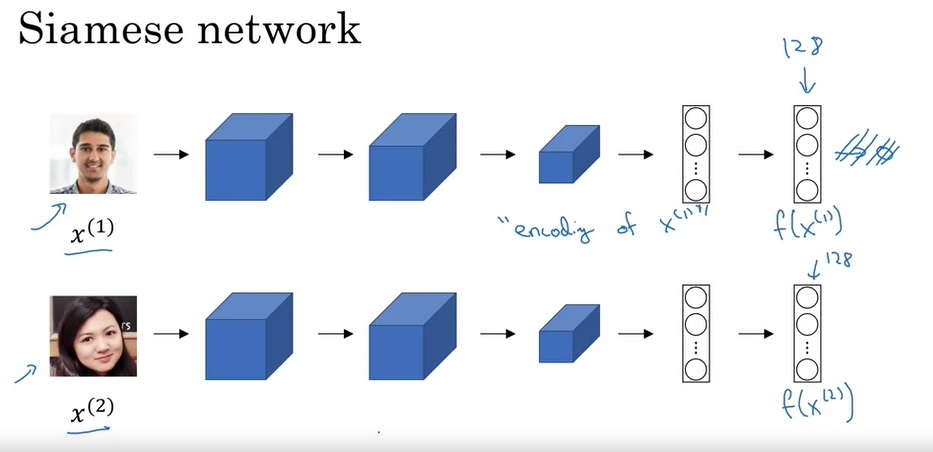• 若$x^{(i)}, x^{(j)}$是同一人，则$||f(x^{(i)}) - f(x^{(j)})||^2$很小。
• 若$x^{(i)}, x^{(j)}$不是同一人，则$||f(x^{(i)}) - f(x^{(j)})||^2$很大。、

### 三元组误差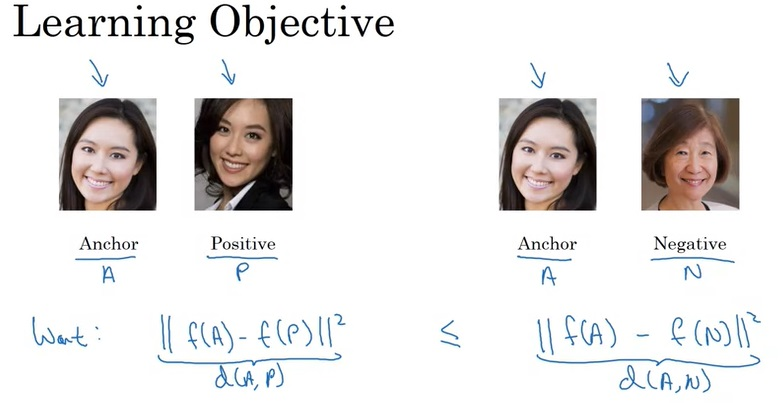## 神经网络风格迁移

### 什么是神经网络风格迁移？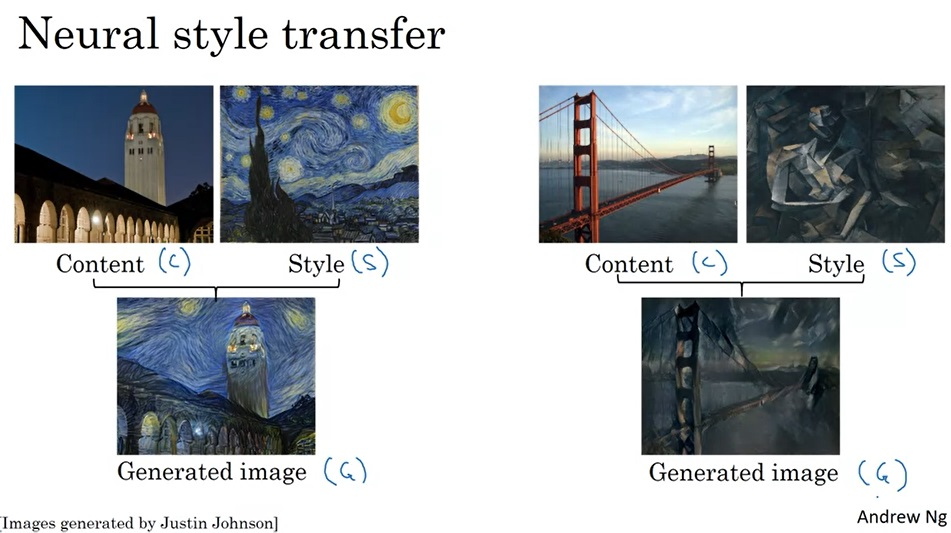### 深度卷积网络学到了什么？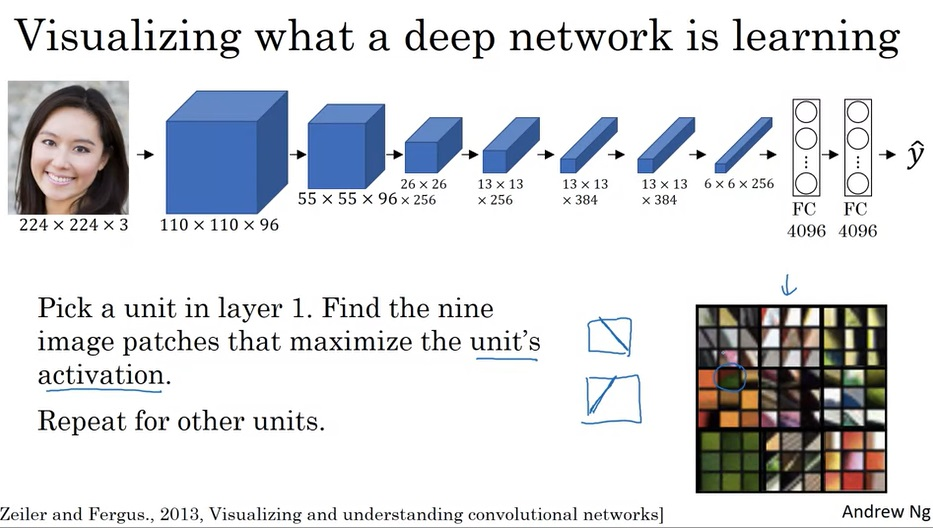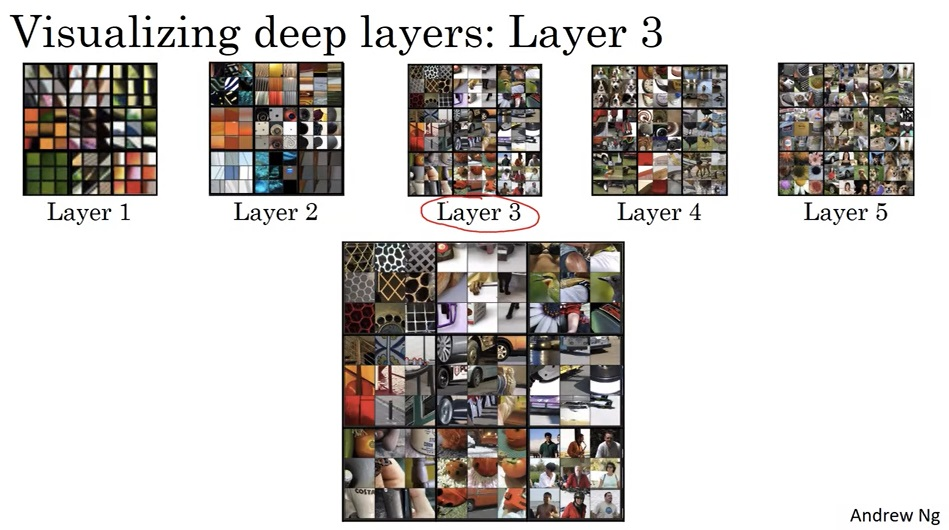### 损失函数

1. 生成一个随机图像$G: 100 \times 100 \times 3$
2. 使用梯度下降更新$G$，最小化$J(G)$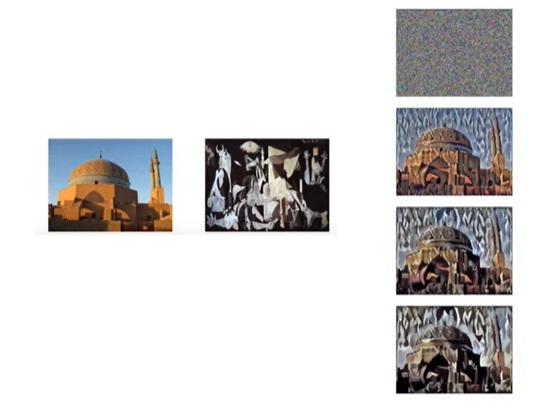### 内容损失

1. 使用一个预训练的网络（最常用的是VGG）。
2. 选择神经网络中的某一层$l$，这一层相关的数据会用来计算内容损失。$l$越浅，表示越具体的图像；$l$越深，表示越抽象的图像。一般选取适中的$l$。
3. 令$a^{l}, a^{[l][G]}$为图像$C, G$网络第$l$层的激活输出。我们认为，如果这两个值很相似，则两幅图像的内容就很相似。
4. 令$J_{content}(C, G) = ||a^{l} - a^{[l][G]}||^2$

### 风格损失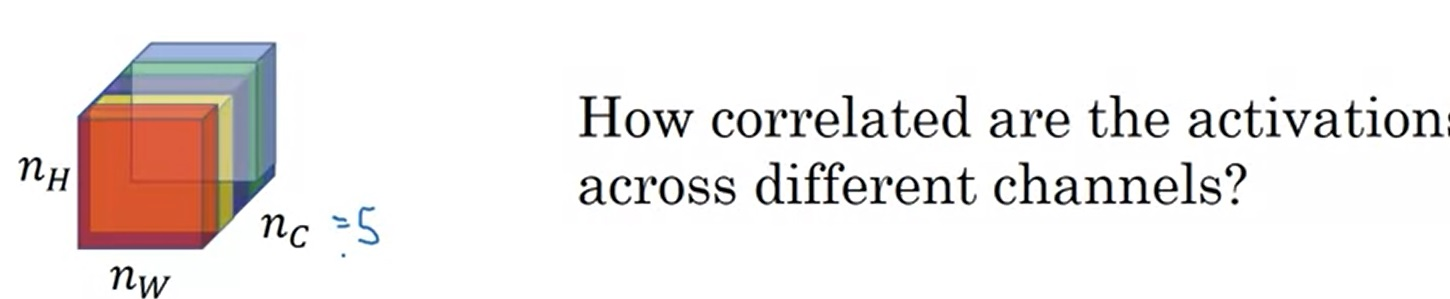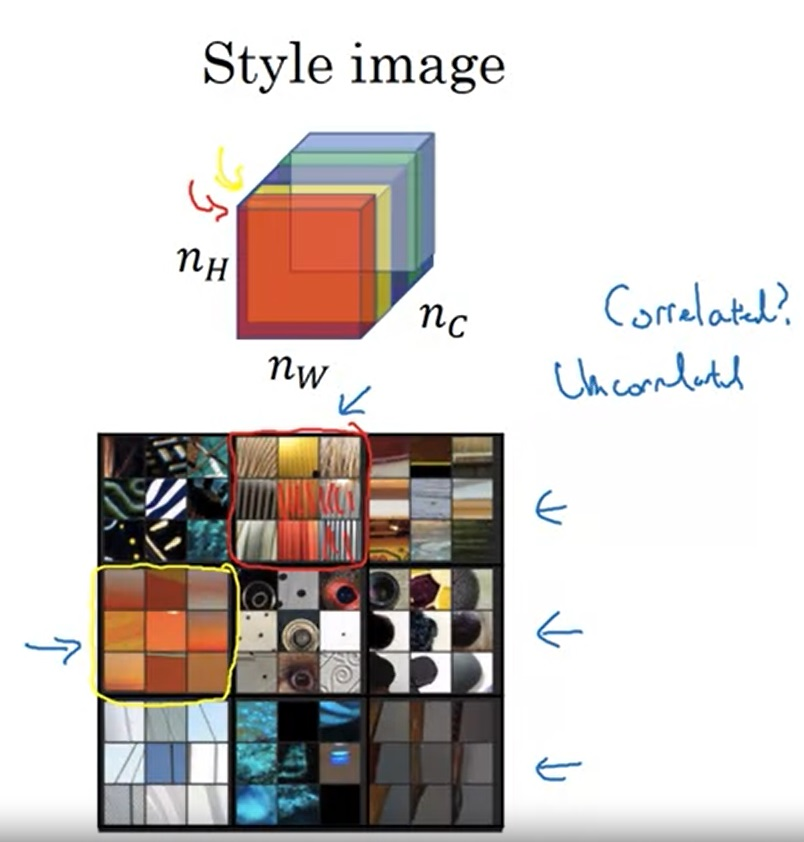## 1D和3D上的卷积

1D数据可以是心电图。和可以用2D卷积捕捉2D图像的边缘一样，我们可以用下图中那个尖尖的1D卷积来捕获高频的数据。1D卷积前后的形状变化规律和2D一样，比如用16个长度为5的卷积卷一个$14 \times 1$的1D图像，可以得到$10 \times 16$的1D图像（第一维是图像长度，第二维是通道数）。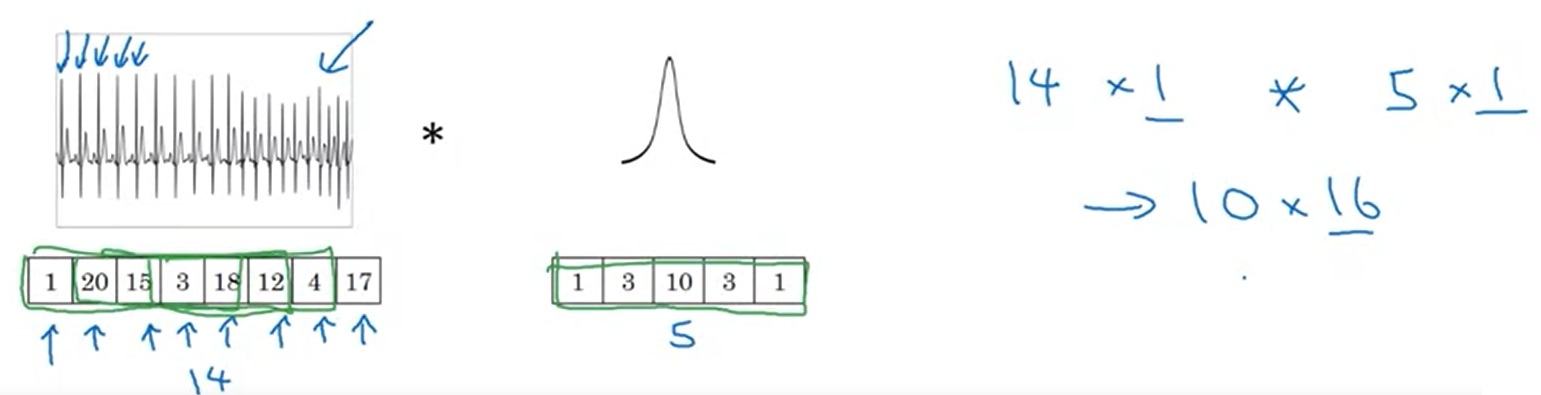3D数据也是类似的道理。用16个$5 \times 5 \times 5$的卷积核卷一个单通道$14 \times 14 \times 14$的单通道图像，可以得到一个$5 \times 5 \times 5 \times 16$的图像。

# 总结

• 人脸识别
• 人脸验证与人脸识别任务的定义
• 如何对单样本学习建模
• 孪生网络
• 基于三元组误差和二分类误差的人脸识别网络
• 神经网络风格迁移
• 神经网络风格迁移的输入输出
• 利用小技巧可视化 CNN 学到的图像信息
• 生成风格迁移图像时的内容误差与风格误差